Friday , October 7 2022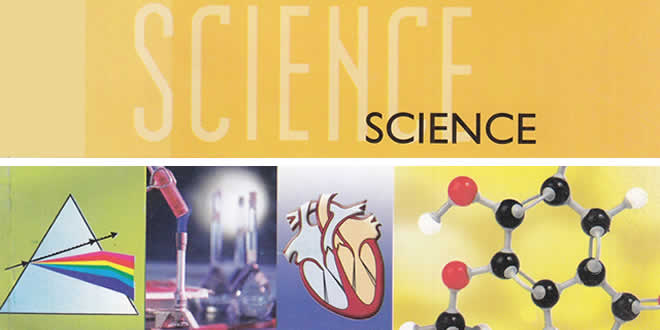# Light Reflection and Refraction: 10th Science Chapter 10

 Class: 10th Class Subject: Science Chapter: Chapter 10: Light Reflection and Refraction Quiz: – Questions MCQs: 95 Questions

## Light Reflection and Refraction

### QA for Class 10 Science Light Reflection and Refraction with Answers & Explanation:

Along with NCERT Solutions For Class 10th Science Chapter 10 Light Reflection and Refraction candidates can also find light reflection and refraction class 10 numerical questions in this article. Go through them will help candidates get a clear idea about how to approach the problems which in turn helps you to solve them in the most efficient way. So why wait? Read on to find out everything about light reflection and refraction class 10 important questions with answers here.

## NCERT Solutions for Class 10 Science Chapter 10

#### Question: Define the principal focus of a concave mirror.

Answer: The principal focus of a concave mirror is a point on its principal axis to which all the light rays which are parallel and close to the axis, converge after reflection from the concave mirror.

#### Question: The radius of curvature of a spherical mirror is 20 cm. What is its focal length?

Answer: Focal length = $$\frac { 1 }{ 2 }$$ x Radius of curvature = $$\frac { 1 }{ 2 }$$ x 20 cm = 10 cm

#### Question: Why do we prefer a convex mirror as a rear-view mirror in vehicles?

Answer: We prefer a convex mirror as a rear-view mirror in vehicles because of two reasons :

1. A convex mirror always produces an erect image of the objects.
2. The image formed in a convex mirror is highly diminished or much smaller than the object, due to which a convex mirror gives a wide field of view of the traffic behind. A convex mirror enables the driver to view such larger area of the traffic behind him.

#### Light Reflection and Refraction – Question: Find the focal length of a convex mirror whose radius of curvature is 32 cm.

Solution: R = +32 cm and $$f=\frac { R }{ 2 } =+\frac { 32 }{ 2 } =+16cm$$

#### Question: A concave mirror produces three times magnified (enlarged) real image of an object placed at 10 cm in front of it. Where is the image located?

Solution: Because the image is real, so magnification m must be negative.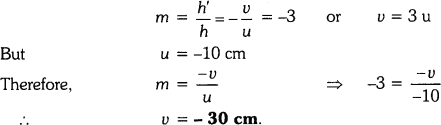Thus the image is located at a distance of 30 cm from the mirror on the object side of the mirror.

#### Question: A ray of light travelling in air enters obliquely into water. Does the light ray bend towards the normal or away from the normal? Why?

Light Reflection and Refraction –  Answer: The light-ray bends towards the normal because the ray of light goes from a rarer medium to a denser medium.

#### Question: Light enters from air to glass having refractive index 1.50. What is the speed of light in the glass? The speed of light in vacuum is 3 x 108 ms-1.

Answer: Refractive index of glass, n8 = 1.50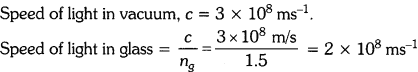#### Question: Find out, from Table 10.3, the medium having highest optical density. Also find the medium with lowest optical density.

Answer: From table 10.3, diamond has highest refractive index (= 2.42), so it has highest optical density.

Air has lowest refractive index (= 1.0003),

so it has lowest optical density.

#### Question: You are given kerosene, turpentine and water. In which of these does the light travel fastest? Use the information given in Table 10.3.

Answer: For kerosene, n = 1.44

For turpentine, n = 1.47

For water, n = 1.33

Because water has the lowest refractive index, therefore light travels fastest in this optically rarer medium than kerosene and turpentine oil.

#### Question: The refractive index of diamond is 2.42. What is the meaning of this statement?

Answer: By saying that the refractive index of diamond is 2.42, we mean that the speed of light in diamond is lower by a factor of 2.42 relative to that in vacuum.

#### Question: Define 1 dioptre of power of a lens.

Answer: One dioptre is the power of a lens whose focal length is 1 metre.

#### Question: A convex lens forms a real and inverted image of a needle at a distance of 50 cm from it. Where is the needle placed in front of the convex lens if the image is equal to the size of the object ? Also, find the power of the lens., Sol. Here, u — +50 cm.

Answer: Here ν = +50cm

Because the real image is of the same size as the object,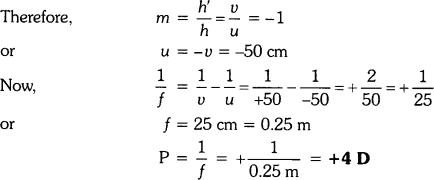#### Question: Find the power of a concave lens of focal length 2 m.

Answer: Because the focal length of a concave lens is negative,

therefore f = -2 m## Light Reflection and Refraction: Textbook Chapter End Questions

(a) Water
(b) Glass
(c) Plastic
(d) Clay

#### Question: The image formed by a concave mirror is observed to be virtual, erect and larger than the object. Where should be the position of the object?

(a) Between the principal focus and the centre of curvature
(b) At the centre of curvature
(c) Beyond the centre of curvature
(d) Between the pole of the mirror and its principal focus

Answer: (d) Between the pole of the mirror and its principal focus

#### Question: Where should an object be placed in front of a convex lens to get a real image of the size of the object ?

(a) At the principal focus of the lens
(b) At twice the focal length
(c) At infinity
(d) Between the optical centre of the lens and its principal focus

Answer: (b) At twice the focal length

#### Question: A spherical mirror and a thin spherical lens have each a focal length of -15 cm. The mirror and the lens are likely to be:

(a) Both concave
(b) Both convex
(c) the mirror is concave and the lens is convex
(d) the mirror is convex, but the lens is concave

Answer: (a) Both concave

#### Question: No matter how far you stand from mirror, your image appears erect. The mirror is likely to be

(a) plane
(b) concave
(c) convex
(d) either plane or convex.

Answer: (d) Either plane or convex.

#### Question: Which of the following lenses would you prefer to use while reading small letters found in a dictionary?

(a) A convex lens of focal length 50 cm
(b) A concave lens of focal length 50 cm
(c) A convex lens of focal length 5 cm
(d) A concave lens of focal length 5 cm

Answer: (c) A convex lens of focal length 5 cm.

#### Question: We wish to obtain an erect image of an object, using a concave mirror of focal length 15 cm. What should be the range of distance of the object from the mirror? What is the nature of the image? Is the image larger or smaller than the object? Draw a ray diagram to show the image formation in this case.

Answer: A concave mirror gives an erect image when the object is placed between the focus F and the pole P of the concave mirror, i.e., between 0 and 15 cm from the mirror. The image thus formed will be virtual, erect and larger than the object.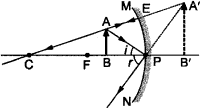#### Question: Name the type of mirror used in the following situations.

(a) Headlights of a car
(b) Side / rear-view mirror of a vehicle
(c) Solar furnace

(a) Concave mirrors are used as reflectors in headlights of cars. When a bulb is located at the focus of the concave mirror, the light rays after reflection from the mirror travel over a large distance as a parallel beam of high intensity.

(b) A convex mirror is used as a side / rear-view mirror of a vehicle because

• A convex mirror always forms an erect, virtual and diminished image of an object placed anywhere in front it.
• A convex mirror has a wider field of view than a plane mirror of the same size.

(c) Large concave mirrors are used to concentrate sunlight to produce heat in solar furnaces.

#### Question: One-half of a convex lens is covered with a black paper. Will this lens produce a complete image of the object ? Verify your answer experimentally. Explain your observations.

Answer: A convex lens forms complete image of an object, even if its one half is covered with black paper. It can be explained by considering following two cases.

Case I: When the upper half of the lens is covered

In this case, a ray of light coming from the object will be refracted by the lower half of the lens. These rays meet at the other side of the lens to form the image of the given object, as shown in the following figure.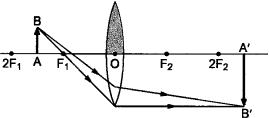Case II: When the lower half of the lens Is covered

In this case, a ray of light coming from the object is refracted by the upper half of the lens. These rays meet at the other side of the lens to form the image of the given object, as shown in the given figure.#### Question: An object 5 cm in length is held 25 cm away from a converging lens of focal length 10 cm. Draw the ray diagram and find the position, size and the nature of the image formed.

Here: Object distance, u= -25 cm,
Object height, h = 5 cm,
Focal length, f = +10 cm
According to the lens formula, $$\frac { 1 }{ f } =\frac { 1 }{ \nu } -\frac { 1 }{ u }$$ , we have
⇒ $$\frac { 1 }{ \nu } =\frac { 1 }{ f } -\frac { 1 }{ u } =\frac { 1 }{ 10 } -\frac { 1 }{ 25 } =\frac { 15 }{ 250 } \quad or\quad \nu =\frac { 250 }{ 15 } =16.66cm$$

The positive value of v shows that the image is formed at the other side of the lens.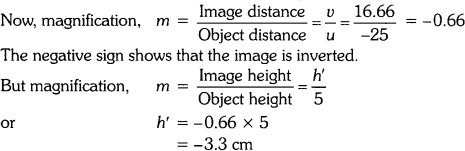The negative value of image height indicates that the image formed is inverted.

The position, size, and nature of image are shown alongside in the ray diagram.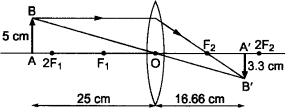#### Question: A concave lens of focal length 15 cm forms an image 10 cm from the lens. How far is the object placed from the lens? Draw the ray diagram.

Answer: Focal length, f = -15 cm, Image distance, ν = -10 cm (as concave lens forms the image on the same side of the lens)

From the lens formula $$\frac { 1 }{ f } =\frac { 1 }{ \nu } -\frac { 1 }{ u }$$ , we have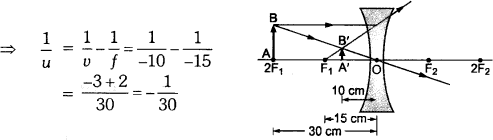Object distance, u = -30 cm

The negative value of u indicates that the object is placed in front of the lens.

#### Question: An object is placed at a distance of 10 cm from a convex mirror of focal length 15 cm. Find the position and nature of the image.

Solution: Object distance, u = -10 cm, Focal length, f = +15 cm, Image distance, ν = ?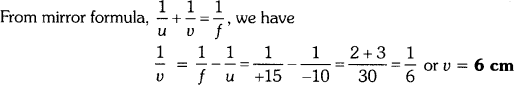Thus, image distance, ν = + 6 cm

Because ν is +ve, so a virtual image is formed at a distance of 6 cm behind the mirror.

Magnification, $$m=\frac { -\upsilon }{ u } =\frac { -6 }{ -30 } =\frac { 1 }{ 5 }$$ (i.e. < 1)

The positive value of m shows that image erect and its value, which is less than 1, shows that image is smaller than the object. Thus, image is virtual, erect and diminished.

#### Light Reflection and Refraction – Question: The magnification produced by a plane mirror is +1. What does this mean?

Answer: Since magnification, $$m=\frac { { h }^{ ‘ } }{ h } =\frac { -\nu }{ u }$$. Given, m = +1, so h’ = h and ν = -u

1. m = 1 indicates the size of image is same as that of object.
2. positive sign of m indicates that an erect image is formed.

The opposite signs of ν and u indicate that image is formed on the other side of the mirror from where the object is placed i.e., image is formed behind the mirror and thus image formed is virtual.

#### Question: An object 5.0 cm in length is placed at a distance of 20 cm in front of a convex mirror of radius of curvature 30 cm. Find the position of the image, its nature and size.

Since object size, h = +5 cm,

object distance, u = -20 cm

and radius of curvature, R = +30 cm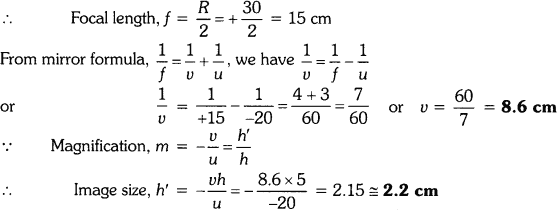A virtual, erect image of height 2.2 cm is formed behind the mirror at a distance of 8.6 cm from the mirror.

#### Question: An object of size 7.0 cm is placed at 27 cm in front of a concave mirror of focal length 18 cm. At what distance from the mirror should a screen be placed, so that a sharp focused image can be obtained ? Find the size and the nature of the image.

Here, object size, h = +7.0 cm,

object distance, u = -27 cm

and focal length, f = -18 cm

Image distance, ν = ?

and image size, h’ = ?

From the mirror formula, $$\frac { 1 }{ f } =\frac { 1 }{ \nu } -\frac { 1 }{ u }$$, we have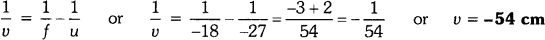The screen should be placed at a distance of 54 cm on the object side of the mirror to obtain a sharp image.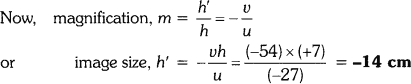The image is real, inverted and enlarged in size.

#### Question: Find the focal length of a lens of power -2.0 D. What type of lens is this?

Here, P = -2.0 D

The type of lens is concave because the focal length is negative.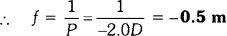#### Question: A doctor has prescribed a corrective lens of power +1.5 D. Find the focal length of the lens. Is the prescribed lens diverging or converging?

Here, P = +1.5 D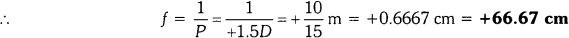Because the focal length is positive, the prescribed lens is converging.

#### Question: Define the principal focus of a concave mirror?

Answer: Light rays that are parallel to the principal axis of a concave mirror converge at a specific point on its principal axis after reflecting from the mirror. This point is known as the principal focus of the concave mirror.

#### Question: The radius of curvature of a spherical mirror is 20 cm. What is its focal length?

Answer: Radius of curvature, R = 20 cm

Radius of curvature of a spherical mirror = 2 x Focal length (f)

f = R/2 = 20/2 =10cm

#### Question: Name the mirror that can give an erect and enlarged image of an object.

Answer: When an object is placed between the pole and the principal focus of a concave mirror, the image formed is virtual, erect, and enlarged.

#### Light Reflection and Refraction – Question: Why do we prefer a convex mirror as a rear-view mirror in vehicles?

Answer: Convex mirrors give a virtual, erect, and diminished image of the objects placed in front of them. They are preferred as a rear-view mirror in vehicles because they give a wider field of view, which allows the driver to see most of the traffic behind him.

#### Question: Find the focal length of a convex mirror whose radius of curvature is 32 cm.

Radius of curvature, R = 32 cm

Radius of curvature = 2 x Focal length (f)

R = 2f

f = R/2 = 32/2 = 16cm

Hence, the focal length of the given convex mirror is 16 cm.

#### Question: A concave mirror produces three times magnified (enlarged) real image of object placed at 10 cm in front of it. Where is the image located?

Given, u = – 10 cm
Since image is real inverted so, m = -3
m = -v / u
⇒ -3 = -v/ -10
v= – 30 cm
Negative sign indicates the image will be real and image is formed at 30 cm in front of the mirror.

#### Question: A ray of light travelling in air enters obliquely into water. Does the light ray bend towards the normal or away from the normal? Why?

Answer: The light ray bends towards the normal. When a ray of light travels from an optically rarer medium to an optically denser medium, it gets bent towards the normal. Since water is optically denser than air, a ray of light travelling from air into the water will bend towards the normal.

#### Question: Light enters from air to glass having refractive index 1.50. What is the speed of light in the glass? The speed of light in vacuum is 3 × 108 m/s.

Refractive index of a medium nm is given by,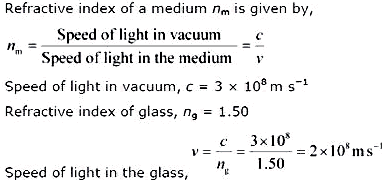#### Question: Find out, from Table, the medium having highest optical density. Also find the medium with lowest optical density.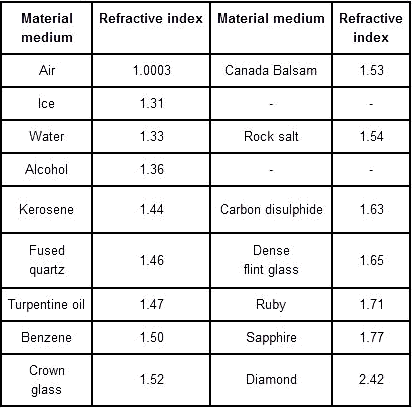Highest optical density = Diamond

Lowest optical density = Air

Optical density of a medium is directly related with the refractive index of that medium. A medium which has the highest refractive index will have the highest optical density and vice-versa.

It can be observed from table 10.3 that diamond and air respectively have the highest and lowest refractive index. Therefore, diamond has the highest optical density and air has the lowest optical density

#### Question: You are given kerosene, turpentine and water. In which of these does the light travel fastest?The light can travel fast through water.

#### Question: The refractive index of diamond is 2.42. What is the meaning of this statement?

Answer: Refractive index of a medium nm is related to the speed of light in that medium v by the relation: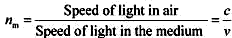Where, c is the speed of light in vacuum/air

The refractive index of diamond is 2.42. This suggests that the speed of light in diamond will reduce by a factor 2.42 compared to its speed in air.

#### Question: Define one dioptre of power of a lens?

One dioptre is the power Of a lens Of focal length 1m.

Power of lens is defined as the reciprocal of its focal length. If P is the power of a lens of focal length F in metres, then

P = 1/ f (in meters)

The S.I. unit of power of a lens is Dioptre. It is denoted by D.

1 dioptre is defined as the power of a lens of focal length 1 metre.

1 D = 1 m−1

#### Question: A convex lens forms a real and inverted image of a needle at a distance of 50 cm from it. Where is the needle placed in front of the lens if the image is equal to the size of the object? Also find the power of the lens.

v = + 50 cm

Since image is real and of same size. The position of image should be double the focal length.

Hence, the object should be at 2f.

V = 2f = 50, f = 25 cm.

Power = 1/f = 100/25 = 4D

#### Light Reflection and Refraction – Question: Find the power of a concave lens of focal length 2 m.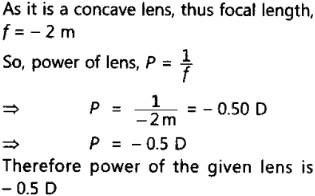(a) Water
(b) Glass
(c) Plastic
(d) Clay

#### Question: The image formed by a concave mirror is observed to be virtual, erect and larger than the object. Where should be the position of the object?

(a) Between the principal focus and the centre of Curvature
(b) At the centre of curvature
(c) Beyond the centre of curvature
(d) Between the pole of the mirror and Its principal focus

Answer: (d) Between the pole of the mirror and its principal focus

#### Question: Where should an object be placed in front of a convex lens to get a real image of the size of the object?

(a) At the principal focus of the lens
(b) At twice the focal length
(c) At infinity
(d) Between the optical centre of the lens and its principal focus

Answer: (b) At twice the focal length

#### Question: A spherical mirror and a thin spherical lens have each a focal length of 15 cm. The mirror and the lens are likely to be:

(a) both concave
(b) both convex
(c) the mirror is concave, but the lens is convex
(d) the mirror is convex, but the lens is concave

Answer: (a) Both concave.

#### Light Reflection and Refraction – Question: No matter how far you stand from a mirror, your Image appears erect. The mirror is likely to be

(a) plane
(b) concave
(c) convex
(d) Either plane or convex

Answer: (d) Either plane or convex.

#### Question: Which of the following lenses would you prefer to use while reading small letters found in a dictionary?

(a) A convex lens of focal length 50 cm
(b) A concave lens of focal length 50 cm
(c) A convex lens of focal length 5 cm
(d) A concave lens of focal length 5 cm

Answer: (c) A convex lens of focal length 5 cm

#### Question: We wish to obtain an erect image of an object, using a concave mirror of focal length 15 cm. what should be the range of distance of the object from the mirror? What is the nature of the image? Is the image larger or smaller than the object? Draw a ray diagram to show the image formation in this case.

Answer: We are given the focal length cf the concave mirror as f = -15cm.
For getting an erect image using a concave mirror, the object should be placed at a distance less than the focal length.
i.e. 15 cm from the pole. The image formed will be virtual, enlarged and erect.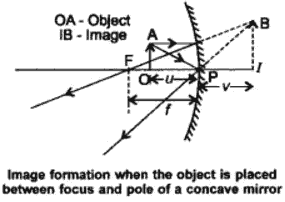#### Light Reflection and Refraction – Question: Name the type of mirror used in the following situations.

(a) Headlights of a car
(b) Side / rear-view mirror of a vehicle
(c) Solar furnace

(a) Concave mirror, to get powerful and parallel beams of light.
(b) Convex mirror because it always gives an erect image and enables the driver to view much larger area.
(c) Concave or parabolic mirror because it can concentrate sunlight at the focus to produce heat in the solar furnace.

#### Question: One half of a convex lens is covered with a black paper. Will this lens produce a complete image of the object? Verify your answer experimentally. Explain your observations.

Answer: Yes, even when one half of the lens is covered with a black paper, complete image of the object will be formed. Take a convex lens and focus the light from a distant object onto a screen. As expected an image (sharp) is formed at a distance equal to the focal length Cover the lower or the upper half of the lens and focus the light from the same object onto the same screen. You will be able to get a sharp image again; however the brightness of the image will be less in the second case. The same effect w,ll be seen even if the lens is half covered with black strips.

#### Question: An object 5cm in length is held 25 cm away from a converging lens of focal length 10 cm. Draw a ray diagram and find the position, size and the nature of the image formed.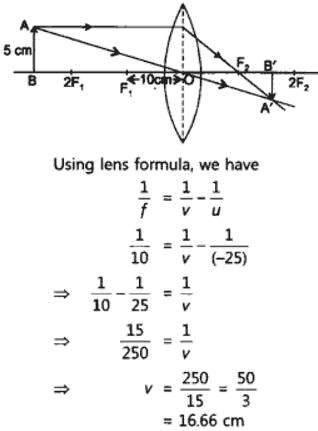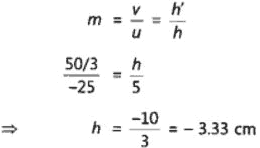Therefore, the mage ¡s formed between F2 and 2F2 on the other side of the lens. It is real and inverted, and smaller in size than the object.

#### Question: A concave lens of focal length 15 cm forms an ¡mage 10 cm from the lens. How far is the object placed from the Pens? Draw the ray diagram.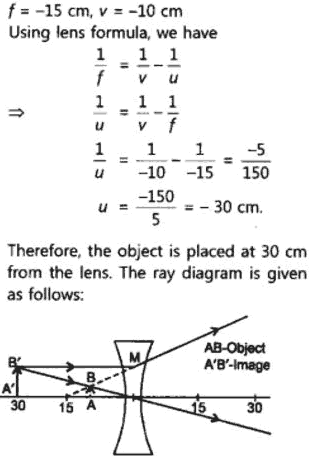#### Question: An object is placed at a distance of 10 cm from a convex mirror of focal length 15 cm. Find the position and nature of the image.

Answer: f = +15 cm. u = -1o cm

For mirror, we have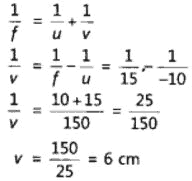The image must be virtual and erect.

#### Light Reflection and Refraction – Question: The magnification produced by a plane mirror is +1. What does this mean?

Answer: This means that size of the image is equal to the size of the object.

#### Question: An object 5.0 cm in length is placed at a distance of 20 cm in front of a convex mirror of radius of curvature 30 cm. Find the position of the image nature and size.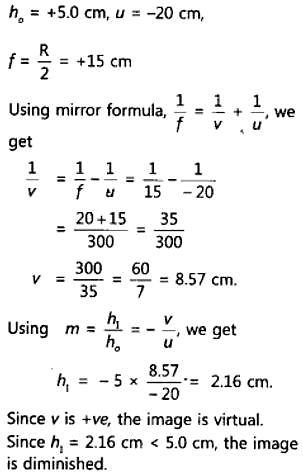#### Question: An object of size 7.0 cm is placed at 27 cm in front of a concave mirror of focal length 18 cm. At what distance from the mirror should a screen be placed, so that a sharp focused image can be obtained? Find the size and the nature of the image.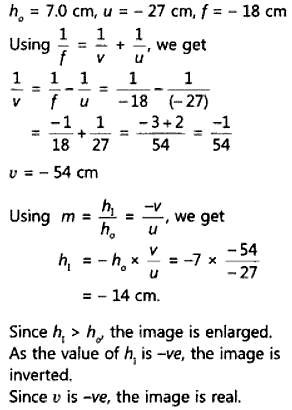#### Question: Find the focal length of a lens of power -2.0 D. What type of lens is this?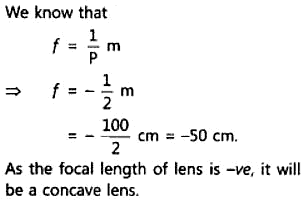#### Question: A doctor has prescribed a corrective lens of power +1.5 D. find the focal length of the lens. Is the prescribed lens diverging or converging?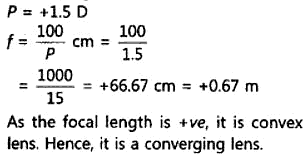### Multiple Choice Questions (MCQs) [1 mark each]

#### Question: Hold a highly polished steel spoon curved inwards close to your face and move it slowly away from your face. What will you observe?

(a) Enlarged and erect image of your face
(b) Smaller and inverted image of your face
(c) Smaller and erect image of your face
(d) Enlarged and inverted image of your face

Answer: (b) The inner curved surface of a highly polished steel spoon acts as a concave mirror. When the spoon is at a small distance from the face such that, the object lies between pole and focus of concave mirror, so an enlarged and erect image of your face will be observed but as the spoon is slowly moved away from the face, the image becomes smaller and appears inverted.

#### Question: Which one of the following materials cannot be used to make a lens? [NCERT]

(a) Water
(b) Glass
(c) Plastic
(d) Clay

Answer: (d) Clay can never be transparent, so it cannot be used to make lens.

#### Question: No matter how far you stand from a mirror, your image appears erect. The mirror is likely to be [NCERT]

(a) plane
(b) concave
(c) convex
(d) either plane or convex

Answer: (d) Plane mirrors and convex mirrors always form the erect images.

#### Question: The image formed by a concave mirror is observed to be virtual, erect and larger than the object. Where should be the position of the object? [NCERT]

(a) Between principal focus and centre of curvature
(b) At centre of curvature
(c) Beyond centre of curvature
(d) Between pole of the mirror and its principal focus

#### Question: An object AB is placed in front of a convex lens at its centre of curvature as shown in figure below.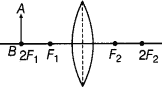####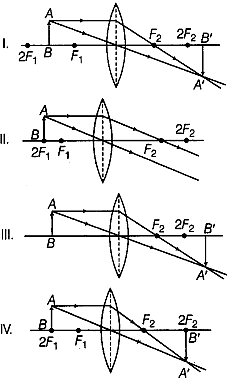(a) Only I
(b) Only II
(c) Only III
(d) Only IV

Answer: (d) When the object is placed at centre of curvature (2Fx) of convex lens, the same sized image is formed at 2F2. The image formed is real and inverted.

#### Question: A spherical mirror and a thin spherical lens have each of a focal length -15 cm. The mirror and lens are likely to be [NCERT]

(a) both concave
(b) both convex
(c) mirror is concave and lens is convex
(d) mirror is convex and lens is concave

Answer: (a) The focal length is taken as negative for both concave mirror and concave lens.

#### Question: Which of the following can make a parallel beam of light when light from a point source is incident on it? [NCERT Exemplar]

(a) Concave mirror as well as convex lens
(b) Convex mirror as well as concave lens
(c) Two plane mirrors placed at 90° to each other
(d) Concave mirror as well as concave lens

Answer: (a) A ray passing through the principal focus of a concave mirror or convex lens, after reflection/refraction, will emerge parallel to the principal axis.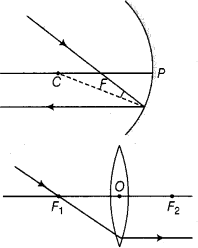#### Question: Under which of the following conditions, a concave mirror can form an image larger than the actual object? [NCERT Exemplar]

(a) When an object is kept at a distance equal to its radius of curvature
(b) When an object is kept at a distance less than its focal length
(c) When an object is placed between the focus and centre of curvature
(d) When an object is kept at a distance greater than its radius of curvature

Answer: (c) A concave mirror can form an image enlarged, real and inverted than the actual object, beyond centre of curvature (C) when object is placed between the focus (F) and centre of curvature.

####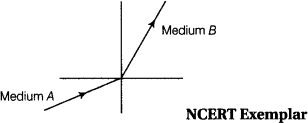(a) greater than unity
(b) less than unity
(c) equal to unity
(d) zero

Answer: (a) Since, light rays in the medium B goes towards normal. So, it has greater refractive index and lesser velocity of light w.r.t. medium A. So, refractive index of medium B w.r.t. medium A is greater than unity.

#### Question: Figure shows a ray of light as it travels from medium A to medium B. Refractive index of the medium B relative to medium A is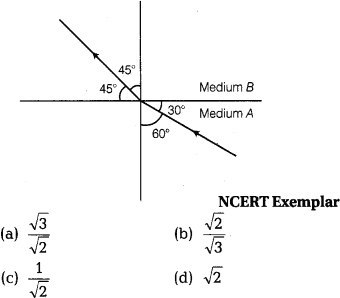Answer: (a) Given, angle of incidence, i = 60°, angle of refraction, r = 45°
Refractive index of the medium B relative to medium A,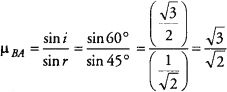#### Question: Beams of light are incident through the holes A and B and emerge out of box through the holes C – and D respectively, as Box shown in the figure.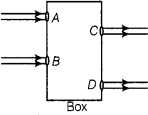#### Which of the following could be inside the box? [NCERT Exemplar]

(a) A rectangular glass slab
(b) A convex lens
(c) A concave lens
(d) A prism

Answer: (a) Here, the emergent rays are parallel to the direction of the incident ray. Therefore, a rectangular glass slab could be inside the box as the extent of bending of light ray at the opposite parallel faces AB (air-glass interface) and CD (glass-air interface) of the rectangular glass slab are equal and opposite. This is why the ray emerges parallel to the incident ray.

#### Question: A beam of light is incident through the holes on side A and emerges out of the holes on the other face of the box as shown in the figure. Which of the following could be inside the box? [NCERT Exemplar]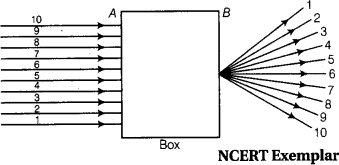(a) Concave lens
(b) Rectangular glass slab
(c) Prism
(d) Convex lens

Answer: (d) Since, in the figure all the parallel rays converge at a point. So, inside the box there must be a convex lens.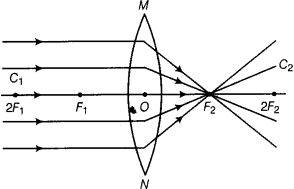#### Question: Which of the following statement is true? [NCERT Exemplar]

(a) A convex lens has 4D power having a focal length 0.25 m
(b) A convex lens has 4D power having a focal length -0.25 m
(c) A concave lens has 4D power having a focal length 0.25 m
(d) A concave lens has 4D power having a focal length -0.25 m

Answer: (a) The power P of a lens of focal length f is given by
P = 1/f, where f is the focal length in metre and P is the power in dioptre.
P= 1/f or f = 1/P = 1/4 = 0.25 m

#### Question: Magnification produced by a rear view mirror fitted in vehicles [NCERT Exemplar]

(a) is less than one
(b) is more than one
(c) is equal to one
(d) can be more than or less than one depending upon the position of the object in front of it.

Answer: (a) The convex mirror forms virtual, erect and diminished image of the object and rear view mirror also form same type of image. Therefore, magnification (m) produced by a rear view mirror fitted in vehicles is less than one, i.e. m < 1.

#### Question: Rays from the Sun converge at a point 15 cm in front of a concave mirror. Where should an object be placed, so that size of its image is equal to the size of the object? [NCERT Exemplar]

(a) 15 cm in front of the mirror
(b) 30 cm in front of the mirror
(c) between 15 cm and 30 cm in front of the mirror
(d) more than 30 cm in front of the mirror

Answer: (b) The rays from the Sun, i.e. from infinity, are parallel to principal axis after reflection converge at a point is known as focus. Therefore, focal length if) of concave mirror is 15 cm. And we know that, same size, real and inverted image is formed by concave mirror when object is placed at focus 2 A or centre of curvature, so to form same size of image, object will be placed at 15 x 2 =30 cm.

#### Question: The path of a ray of light coming from air passing through a rectangular glass slab traced by four students shown as I, II, III and IV in the figure. Which one of them is correct? [NCERT Exemplar]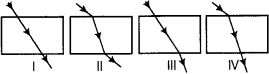(a) Only I
(b) Only II
(c) Only III
(d) Only IV

Light Reflection and Refraction –  Answer: (b) In a rectangular glass slab, the emergent rays are parallel to the direction of the incident ray, because the lateral deviation of bending of the ray of light at the opposite parallel faces (air-glass interface) and (glass-air interface) of the rectangular glass slab are equal and opposite. This is why the ray emerges are parallel to the incident ray.

#### Question: You are given water, mustard oil, glycerin and kerosene. In which of these media, a ray of light incident obliquely at same angle would bend the most? [NCERT Exemplar]

(a) Kerosene
(b) Water
(c) Mustard oil
(d) Glycerin

Answer: (d) The given material having their refractive index as kerosene is 1.44, water is 1.33, mustard oil is 1.46 and glycerin is 1.74. Thus, glycerin is most optically denser and hence have the largest refractive index. Therefore, ray of light bend most in glycerin.

#### Question: A student placed a light bulb in midway between the two plane mirrors inclined at an angle of 60°. How many images will be observed by him?

(a) 4
(b) 6
(c) 5
(d) 8

Light Reflection and Refraction – Answer: (c) Number of images formed by two plane mirrors inclined at an angle 60° when a light bulb is placed in midway between them is
N = 360°/60° – 1 = 6 – 1 = 5

#### Question: Where should an object be placed in front of a convex lens to get a real image of the size of the object? [NCERT]

(a) At the principal focus of the lens
(b) At twice the focal length
(c) At infinity
(d) Between the optical centre of the lens and its principal focus

Answer: (b) To set the real image of the size of the object, it should be placed at twice the focal length of a convex lens.

#### Question: Which of the following lenses would you prefer to use while reading small letters found in dictionary? [NCERT]

(a) A convex lens of focal length 50 cm
(b) A concave lens of focal length 50 cm
(c) A convex lens of focal length 5 cm
(d) A concave lens of focal length 5 cm

Light Reflection and Refraction –  Answer: (c) Convex lens is used as magnifying glass. For better performance its focal length should be small.

## Sources of Energy: 10th Science Chapter 14

Class: 10th Class Subject: Science Chapter: Chapter 14: Sources of Energy Quiz: – Questions MCQs: …